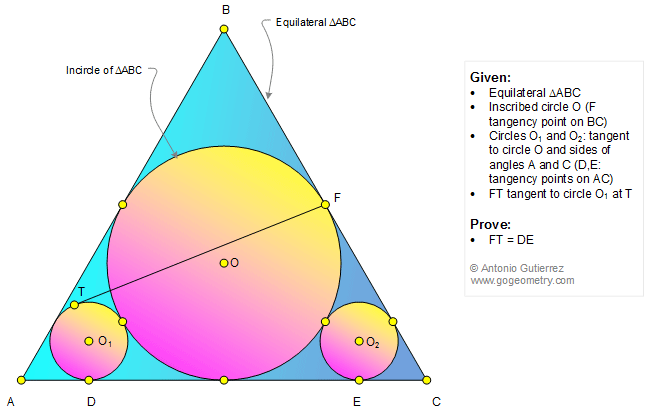# Geometry Problem 1314: Equilateral Triangle, Incircle, Inscribed Circle, Tangent, Congruence. Level: High School, College

< PREVIOUS PROBLEM  |  NEXT PROBLEM >

The figure shows an equilateral triangle ABC with the incircle O (F is the tangency point on BC). Circles O1 and O2 are tangent to circle O and to the sides of angles A and C (D and E are the tangency points on AC). If FT is tangent to circle O1 at T, prove that FT = DE.## Sketch of problem 1314Problems
All Problems
Open Problems
Visual Index
1311-1320
Triangle
Equilateral Triangle
Circle
Incircle
Tangent Circles
Tangent line
Congruence
View or post a solution
Kaleidoscope of problem 1314 using iPad Apps

Related Topics

Home | Geometry | Email
Last updated: Dec 6, 2017